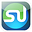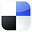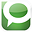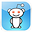# Nonparametric TestsWhen To Use Them in Marketing

Statistical procedures are either parametric or nonparametric. Parametric statistical tests require assumptions about the population from which the samples are drawn. For example, many tests such as the t Test, Chi-Square tests, z Tests, and F tests, and many types of hypothesis tests require the underlying population to be normally distributed. Some tests require equal variances of both populations.

Sometimes these assumptions cannot be always be assumed. Examples of this would be if the population is highly skewed or if the underlying distribution or variances were entirely unknown.

Nonparametric tests have no assumptions regarding distribution of underlying populations or variance. Most of this are very easy to perform but they are not usually as precise as parametric tests and the Null Hypothesis usually requires more evidence to be rejected in a nonparametric test.

# When To Use Nonparametric Tests

Nonparametric tests are often used as shortcut replacements for more complicated parametric tests. You can quite often get a quick answer that requires little calculation by running a nonparametric test.

Nonparametric tests are often used when the data is ranked but cannot be quantified. For example, how would you quantify consumer rankings such as very satisfied, moderately satisfied, just satisfied, less than satisfied, dissatisfied?

Nonparametric tests can be applied when there are a lot of outliers that might skew the results. Nonparametric tests often evaluate medians rather than means and therefore if the data have one or two outliers, the outcome of the analysis is not affected.

They come in especially handy when dealing with non-numeric data, such as having customers rank products or attributes according to preference.

If you would like to create a link to this blog article, here is the link to copy for your convenience:

Nonparametric Tests - When Should the Marketer Use Them?If You Like This, Then Share It...Excel Master Series Blog Directory

Statistical Topics and Articles In Each Topic

• Histograms in Excel
• Bar Chart in Excel
• Combinations & Permutations in Excel
• Normal Distribution in Excel
• t-Distribution in Excel
• Binomial Distribution in Excel
• z-Tests in Excel
• t-Tests in Excel
• Hypothesis Tests of Proportion in Excel
• Chi-Square Independence Tests in Excel
• Chi-Square Goodness-Of-Fit Tests in Excel
• F Tests in Excel
• Correlation in Excel
• Pearson Correlation in Excel
• Spearman Correlation in Excel
• Confidence Intervals in Excel
• Simple Linear Regression in Excel
• Multiple Linear Regression in Excel
• Logistic Regression in Excel
• Single-Factor ANOVA in Excel
• Two-Factor ANOVA With Replication in Excel
• Two-Factor ANOVA Without Replication in Excel
• Creating Interactive Graphs of Statistical Distributions in Excel
• Solving Problems With Other Distributions in Excel
• Optimization With Excel Solver
• Chi-Square Population Variance Test in Excel
• Analyzing Data With Pivot Tables
• SEO Functions in Excel
• Time Series Analysis in Excel

1.Well Said, you have furnished the right information that will be useful to anyone at all time. Thanks for sharing your Ideas.

software testing course in chennai

2.Great info. I love all the posts, I really enjoyed,
nice post and site, good work!